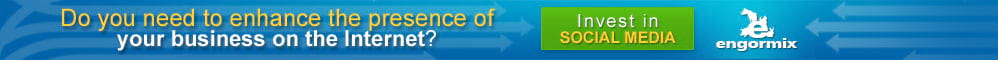# Design and Development of Nutrient Requirement Calculator for Dairy Cattle Feed Formulation

Published on: 1/30/2019
Author/s :
Summary

Linear programming (LP) is one amongst the models that is in use effectively since many years for least cost feed formulation. Newer techniques are available for solving mathematical problem, where the success rate is found to be varying. Hence an attempt was made to develop farmer friendly “Nutrient Requirement Calculator” in VB.NET for dairy cattle to calculate nutrient requirement with appropriate coding. This study involved in three phases: First Design and development of a “Nutrient Requirement Calculator”, secondly development of Linear mathematical model to optimize feed cost using three different techniques such as Random Search Technique (RST), MATLAB and LP in Excel and third translation of results into simpler farmer friendly terms to execute at farm. Result shows that there is no significance difference exists between the methods (P > 0.05) therefore this calculator can be used to calculate the nutrient requirement of cattle’s as per NRC 2001 standards.

Key words: Feed formulation, Linear Programming, Excel Solver, Random Search Technique, MATLAB® toolbox, Nutrient Requirement Calculator.

INTRODUCTION

Feed (ration) formulation is a technique by which different feed ingredients are combined to provide required nutrient to animals for a period of time, usually 24 hours, at different stages of production. A ration should supply all essential nutrients and energy to maintain vital physiological functions of growth, reproduction and health of animals. Ration should be palatable, digestible with permissible level of anti-nutritional factors, economical and should have very less adverse environmental effect.

In (Chakeredza et al, 2008) presented a systematic MS Excel spread sheet based procedure of diet formulation for smallholder dairy production of sub-Saharan Africa. This procedure ensures that the diet is balanced for all the key nutrients, is low-cost and the user has significant control over the formulation process. Using this formulation method, Author incorporated the fodder legumes Leucaena diversifolia, Leucaena pallidaetc, which results in cost reduction from 10% on C. calothyrsus to 30% on L. diversifolia.  In (Pratiksha et al, 2012) optimized animal weight gain using nonlinear programming technique and C language to explores the use of mathematical and computerized programming in the field of animal nutrition and can be investigated in future for more variables. Recently (Goswami et al, 2013) presented a least cost balanced diet formulation plan for small dairy farmers in central India and used Linear programming technique to formulate the diet plan for cross breed dairy cows yielding 5 to 10 lit milk per day included 3.50 kg paddy straw, 10.60 kg Napier grass, 1.35 kg soybean cake, 2.08 kg wheat bran, and 0.06  kg  mineral  mixture,  which cost 19  % less   compare  to routine feed plan. Also they formulated the diet plan for lactating cows yielding 3 Lit milk per day which includes 3.06 kg paddy straw, 7.60  kg Napier  grass, 0.86  kg soybean cake, 1.20  kg wheat bran, and 0.05  kg  mineral  mixture, which cost 22% less compare to existing plan at that time. Similarly, (Onwurah et al, 2011) had developed feed formulation in MS Excel package for birds.(Janzekovic et al, 2014) Developed mathematical model in Whats Best 9 (WB!9) for Excel to optimise a fodder for dairy cows which results in minimizing the feeding ration cost.(Gupta et al, 2013) tried other techniques namely Random Search Technique and Genetic algorithm to formulate the least cost ration for sahiwal cows of second to fifth lactation number to maximize the milk yield based on secondary data. Results obtained shows that the performance of both the techniques can be implemented for nonlinear livestock ration formulation problem. In (Bhagat et al, 2014) formulated the least cost ration for milking Pandharpuri buffalo based on secondary data to formulate the least cost ration and costing 19.99  % less than the routine feeding plan (Ravinder et al, 2016) used ICAR 2013 as well as NRC 2001 standards, to calculate the nutrient bounds to formulate the least cost ration by adopting different types of cattle, weighing 500 kg, 10 L milk yield with 4 % of fat content and at third trimester of pregnancy. Real coded Genetic algorithm was implemented to solve the linear model, which gives least cost of 6 Rupees per kg and requires 30 kg of approximate TMR on Dry Matter basis.

Dairy cattle feed formulation was determined with linear programming for minimizing cost subject to nutrient requirements of particular physiological needs of an animal. This approach allowed the farm manager to make livestock ration decisions based on the prices of feed ingredients and availability of feed. Dairy industries have a variety of feed resources and objectives in which animal ration formulation and nutrient utilization with minimum cost are important one. For example, dairy cows are fed to minimize cost subject to achieving a level of milk production, which, in turn, dictates protein, energy, and vitamin and mineral levels. This method ignores the cost of over-feeding specific nutrients that may accompany the lowest cost of protein and energy sources. The objective of feed formulation is to provide set of feed ingredients to animal, which fulfil the nutrient requirement of animal to exploit its highest genetic potential for its maximum production in the best way. The feed formulation model seeks the optimum combination of available feed ingredients that will satisfy the nutritional requirements of the animal at the least possible cost.

• Dry animals with pregnancy;
• Animals in milk and
• Milch animal with pregnancy.

Feeding schedules observed including feeding of dry roughages including paddy straw and ragi straw as majority, green fodder includes Bajra-Napier grass, CoFS -29 multicut sorghum, Maize fodder etc., and concentrate feed include maize grain, de-oiled cakes (DOC) viz., soya , copra and cotton, and wheat bran, cotton seed, concentrate mixture type I etc., The dairy cows during pregnancy need balanced ration for body maintenance, fetus growth and for milk production (with minimum of 4 % of fat content). Hence preparation of least cost balance feed is challenging to the farmers. In this experiment, 3 case studies depending upon different nutrient requirement of three cattle had been considered. First cattle named as case 1 in which weight of animal is 400kg, age is 4 years, and pregnant, Second cattle named as case 2 in which weight of animal is 400kg, milk yield is 10 litre, fat is 4 %, not pregnant, age is 4 years, Third cow named as case 3 in which weight of animal is 400kg, milk yield is 10 litre, fat is 4 %, age is 4 years. For all the three cases, nutrient requirement was calculated using Nutrient Requirement Calculator and further ration was formulated by three linear models and solved by three different techniques:

The model has to satisfy a set of constraints on nutritional levels, availability restrictions and special ingredients to be included. This study involved in three phases: First Design and development of a “Nutrient Requirement Calculator”, which can calculate the nutrient requirement accurately without violating the nutrient requirements the second development of LP model in Excel Solver, and Controlled Random Search Technique for global optimization and linear programming in MATLAB® toolbox for finding least cost of the ration and third translation of results into simpler farmer friendly terms to execute at farm.

MATERIALS AND METHODS

A survey was carried out at Mandya and Kolar district of Karnataka. About 500 crossbreed cattle were surveyed and weight, feeding schedules and milk yield were collected. It was found that average weight of the animal is about 400 kg and milking animals were three categories Technique for global optimization and linear programming in MATLAB® toolbox. Hence, this paper has three major components database & processing, programming & feed formulation, Solution and output.

Database & Processing

Nutrient composition of feedstuffs is divided into three categories as Roughages, Concentrate & Minerals which is shown in below table with their nutrient composition (Table 1). Parameters for the composition include the dry matter, CP,TDN, Ca, P and cost of the ingredient have been included based on the present rates for the traded commodities and for other resources that are not traded, costs have been provided based on survey. We have included the concentrate mixtures as dairy farmers and livestock owners invariably use concentrate mixtures in ration formulation. A database has been collected from NIANP, Bangalore with various tables in MS-Access and integrated those based on RDBMS concept. (Table 2) shows the upper and lower bound for all 16 feeds.

Programming and Feed Formulation

A VB.NET program has been used to develop Nutrient Requirement Calculator. It is an object oriented programming language which is combination of visual basic and .NET frameworks. Visual basic has several features for development of large and complex application. The .NET framework is a big library of code which is created to reduce the amount of common programming languages.One major challenge that we faced in formulating any optimal model (Linear/Nonlinear) is how to take decision on fixing the lower and upper limits of variables and constraints. One depend totally on experience and expertise of nutritionist to decide these limits and if it does not give the solution then again making some hit & trial methods to fix the bounds. This challenge motivated us to develop a tool box which can automatically calculate these bounds, once the basic information is given as input.This tool box is developed to calculate nutrient requirement of cattle like Dry Matter (DM) intake, Crude Protein (CP), Total Digestible Nutrient (TDN), Calcium (Ca), Phosphorus (P). It needs the minimum information as an input like body weight, milk yield, milk fat, age, status of the animal (Pregnant or Non Pregnant).

After providing all the necessary information properly, one click on ‘COMPUTE’ calculator will display all minimum and maximum bounds. This software is developed using VB.Net programme (details and code is given as annexure 1) and it is in five different languages English, Hindi, Kannada, Marathi, and Telugu, which contain huge data as per NRC 2011 requirement. Steps followed for least cost formulation is given in (Fig.1)

Schematic diagram

Feed Formulation: Linear programming (LP) for feed formulation is formulated for all the three cases. The components of a LP model contain an objective function, decision variables, constraints, and parameters. The main assumptions of an LP program include linearity, divisibility, certainty, and non-negativity. Linear programming has been widely used in the area of optimizing cost performance subject to animal performance and the nutritional requirements that a specified performance level dictates. Different cases are shown in (Table 3)

Depending upon these 3 cases three linear mathematical models are formulated to get least cost ration. In these models mainly seven constraints are included namely Protein, Energy, calcium, Phosphorus, Dry Matter, amount of  roughage, amount of concentrate and feed constraint.

##### Notations

xj: Quantities of feed ingredients

cj: Cost of feed ingredients

cpj : per unit protein content;

ej : represents the per unit energy content;

caj : represents the per unit calcium content;

phj : represents the per unit phosphorus content;

CPmin : the minimum crude protein level;

CPmax : the maximum crude protein level;

Emin : the minimum energy level;

Emax : maximum energy level;

CAmin : minimum calcium level;

CAmax : maximum calcium level;

PHmin : minimum phosphorus level:

PHmax : maximum phosphorus level;

Rgmin : the minimum roughage level;

Rgmax : the maximum roughage level;

Cnmin : the minimum concentrate level;

Cnmax : the maximum concentrate level.

b1i : the upper limit of feed ingredient in case 1;

b2i : the upper limit of feed ingredient in case 2;

b3i : the upper limit of feed ingredient in case 3,

Z : Objective function,

COSTi : Per unit cost of feed ingredient,

Xi : Variables of feed ingredient in the feed mix,

DMi : Per unit dry matter of feed ingredient,

CPi : Per unit crude protein of feed ingredient,

TDNi : Per unit total digestible nutrient of feed ingredient,

CAi : Per unit calcium of feed ingredient,

Pi : Per unit Phosphorus of feed ingredient,

MINi : Minimum requirement of ith nutrient,

MAX1i: Maximum requirement of ith nutrient in case 1,

MAX2i : Maximum requirement of ith nutrient in case 2,

MAX3i : Maximum requirement of ith nutrient in case 3,

All the three LP models are developed on the basis of the (Table 1). The coefficients of the objectives are taken from (Table 1). The minimum and maximum values of feeds and constraint are taken from Table 2 & 4 respectively.

Solution and Output

After providing inputs of the animal details, user proceeds to calculate by clicking on ‘COMPUTE’ icon that gives the desired output with minimum and maximum bounds of CP, CA, P and TDN with their quantities, it is given in (Table 4). The screenshot of nutrient calculator is depicted in (Fig.2), (Fig 3), (Fig.4) with respective all three cases.

RESULTS AND DISCUSSION

Results of all three cases with three different techniques are shown in (Table 5). Most of the least cost feed formulation requires expertise to decide the bounds of constraints accurately to develop a linear programming model.

Dairy farmers to formulate ration they have to depend upon the professionals. Nutrient requirement calculator is specially developed to fulfil these requirements of dairy farmers. Hence professional expert decision making is made simple in the form of Nutrient Requirement Calculator. Nutrient require of the case 1,2 and 3 have been calculated using Nutrient Requirement Calculator. And it was verified with manual calculation and it showed accurate results. It is easy to operate and need minimum information as input data & on single click it will compute bounds accurately. It is farmer friendly as it developed in local language apart from English viz. Kannada, Marathi, Telugu. The result obtained is further used to calculate least cost feed formulation in Linear Programing models. These data are solved by three different techniques those are Excel Solver, Random Search Technique (RST), MATLAB® toolbox.

Ms-Excel (Lp Simplex)

It is most popular and widely available spread sheet software. It is similar to a large number of people, provides a rich environment for solving non-linear program (NLP) problems in a systematic way. MS-EXCEL comes bundled with MS Office and hence no extra cost is involved by the use of this method. In Ms-excel 2010 we can solve the linear program problem (LPP) as well as NLP problems by using solver option. There are different solver options like generalised reduced gradient (GRG) Non-linear, Simplex LP and Evolutionary.

Nutrient requirement calculator was used to obtain required nutrients and bound (Table 4) for formulation of least cost feed formulation using MS- Excel (LP Simplex) under data tab as described by (Rajendran et al, 2015). The results show that Excel LP simplex calculated least cost feed formulation as compared to other (RST and MATLAB) methods. However, results were not significant between the methods. Based on animal physiological status per day feed was calculated and it was calculate first on DM basis as shown in Table 5 and calculated on the basis of as fed basis (fresh basis) with moisture content Table 6. The cost of feed per kg by Excel LP simplex in case 1, 2 and 3 were INR 4.893778, 4.549833 and 5.285919respectively. After arriving least cost ration by simplex LP method the results were statistically compared with results arrived in other methods, RST (Random Search Technique), MATLABon fresh basis (Table 6).

Similarly (Ravinder et al, 2016) calculated for dairy cattle weighing 500kg, yielding 10 lit of milk yield with 4% fat and pregnant about 7 month for Rs.5.83 per Kg of feed and it showed Excel Simplex LP was the least among other method (simplex LP, GRG Non-linear, Evolutionary Algorithm (EA) and RGA with and without seeding the random number generations) compared. However, they have not used RST method for calculation purpose. In this study, it is not possible to compare the rate obtained with earlier studies due to variation in diet composition, price change and method used. It may be concluded that RST method showed non-significantly better result than other methods.

RST (Random Search Technique)

A controlled “Random Search Technique” (RST) for global optimization can solve large class of optimization problems. These methods become challenging in some specific situations like when there is only limited computer memory available, when the function to be minimized is very "bumpy," when it is highly desirable to find the global minimum of a function having many local minima, (Francisco et al, 1981).

In this study results obtained from RST method is the least cost among all other methods (Simplex LP and MATLAB). The feed cost per day in case 1, 2, and 3 was calculated as INR 4.893665, 4.549551 and 5.280403 respectively on as such basis (Table 6) corresponding to total requirement feed cost of INR 4.893778, 4.549833 and 5.285919 respectively. However, results were not significant (P>0.05) with other methods. In any commercial use, slight decrease in cost have high impact if it is applicable for large volume, hence RST method is may be stated as suitable method for further developing software for feed formulation. (Gupta et al, 2013)Had developed a non- linear RST model for dairy cow and concluded that non-linear weighted sum goal programming approach problem for livestock ration formulation well-structured and more acceptable as compared to linear Goal programming problem.

Matlab ("Matrixlaboratory")

It is high performance language; it is a tool for numerical computing. MATLAB includes computation,  visualization, and programming. In this, problems and solutions are expressed in familiar mathematical notation. The toolbox includes solvers for unconstrained and constrained nonlinear optimization and solves for least-squares optimization. Like for Linear and Quadratic Minimization problems linprog and quadprog respectively and for Nonlinear minimization of functions fminbn, fmincon, fminsearch (TU-Ilmenau et al, 2007) General form of linear programming is as follows

Where, A – Matrix of inequality constraints those are (CP, TDN, CA, P, Roughages, Concentrate)

B- Matrix of equality constraints those are (Dry Matter)

a- RHS of inequality constraints b- RHS of equality constraints

c- Coefficient vector of objective (cost of all feeds )

lb- lower bounds of all feeds

ub- Upper bounds of all feeds

For ration formulation we have used Linprog-Linear Programming toolbox. Once toolbox is selected Mat lab displays the fields of the structure options, in which algorithms are being implemented is to be selected. There are three types of algorithms such as simplex algorithm; an active-set algorithm; a primal-dual interior point method (Large scale). The simplex and active-set algorithms are used to solve medium-scale linear programming problems, if it fails to solve then the large scale is used. And here we have used active-set algorithm because our formulation contain 16 variables. Last step is to define the matrices A, B and the vectors c,a,b,lb and ub, and click on the start button it will give least cost formulation.

The result obtained from MATLAB technique is comparable with other methods. The feed cost per day per kg was calculated as INR 4.923659, 4.54979 and 5.285899 for case 1,2 and 3 respectively (Table 7) corresponding total cost per day was calculated as INR 4.893778, 4.549833 and 5.285919, respectively. MATLAB is commercial software and integrating “Nutrient Requirement Calculator” is difficult to make specific feed formulation software for farmers use. However, the results were comparable with other methods; it can be used for customising feed formulation software. Similarly, (Ravinder et al, 2016) had tried to make feed formulation with METLAB using algorithm of GRG Non- linear, Evolutionary, and Real Coded Genetic Algorithm (RGA) with and without seeding the random number generations and concluded that real code genetic algorithm (RGA) method can also be used for ration formulation to find least cost feedstuffs in dairy cattle.

The (Graph.5), (Graph.6) and (Graph.7) shows the values of the all “feedstuffs” of Case 1, Case 2 and Case 3 respectively, and it is observed that there is no significant difference between the inclusion level of feed ingredients in all methods. In the graph x- axis shows the variables and y axis level of inclusion of feeds ingredients.

The (Table 6)shows the quantity of each feeds in all the three techniques and  cost of TMR on as such basis,  for  example   x1 = 1.5643 kg in  terms  of  dry  matter  then  as  fresh  basis x1 = (1.5643 ∗ 100)/Dn of x1= 1.7381  similarly  all  the feeds in terms of as fresh basis were calculated. In Case 1, for a cattle on an average 18.70341 kg of TMR is required which contain all the required nutrients (CP, TDN, Ca and P) and cost will be INR 91.71239. In Case 2 for a cattle on an average 35.54555 kg of TMR is required which contain all the required nutrients (CP, TDN, Ca and P) and cost will be INR 161.7225. In Case 3 for cattle on an average 35.54464 kg of TMR is required which contain all required nutrients as mentioned and cost will be INR 187.8207.

(Table 7) shows that the cost of ration per cattle in INR on “as fresh basis” by all techniques. One-way ANOVA test at 5% level of significance has been performed for the “Null hypothesis: there is no significant difference between techniques”. The test shows that since p value is greater than 0.05, there is no significance difference between the techniques. Hence, it is proved that nutrient bounds calculated by “Nutrient Requirement Calculator” can be used in linear models to find least cost ration formulation.

CONCLUSION

The main purpose of this study is to develop a farmer friendly “Nutrient Requirement Calculator” in VB.NET which is used to calculate the nutrient requirement of cattle by fixing a minimum and maximum range of feedstuffs. By this bound requirements three linear programming models are formed and solved by three different techniques i.e. Random Search Technique (RST), MATLAB and LP in Excel on dry matter and as fresh basis. Results obtained are significantly similar see (Table 5) and (Table 6). It is concluded that developed nutrient requirement calculator in VB.Net is well accepted as this calculator is capable of finding correct nutrient requirement like minimum and maximum of feeds ingredients constraints, total DM, CP, TDN, Ca and P requirement to overcome the limitation of variations in nutrients, also these values can be used to formulate least cost linear models and can be solved by any suitable methods which gives optimal results without constraints violation. In future this Nutrient Requirement Calculator can be further used for other least cost feed formulation software with some improvement in coding.

Bibliographic references

Author/s

remove_red_eye 1077 forum 2 bar_chart Statistics share print
Share :
close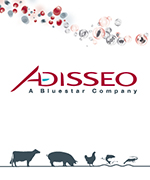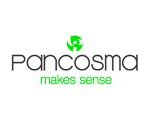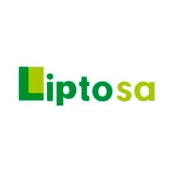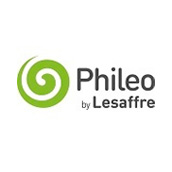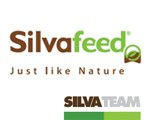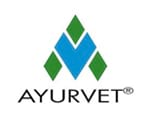|